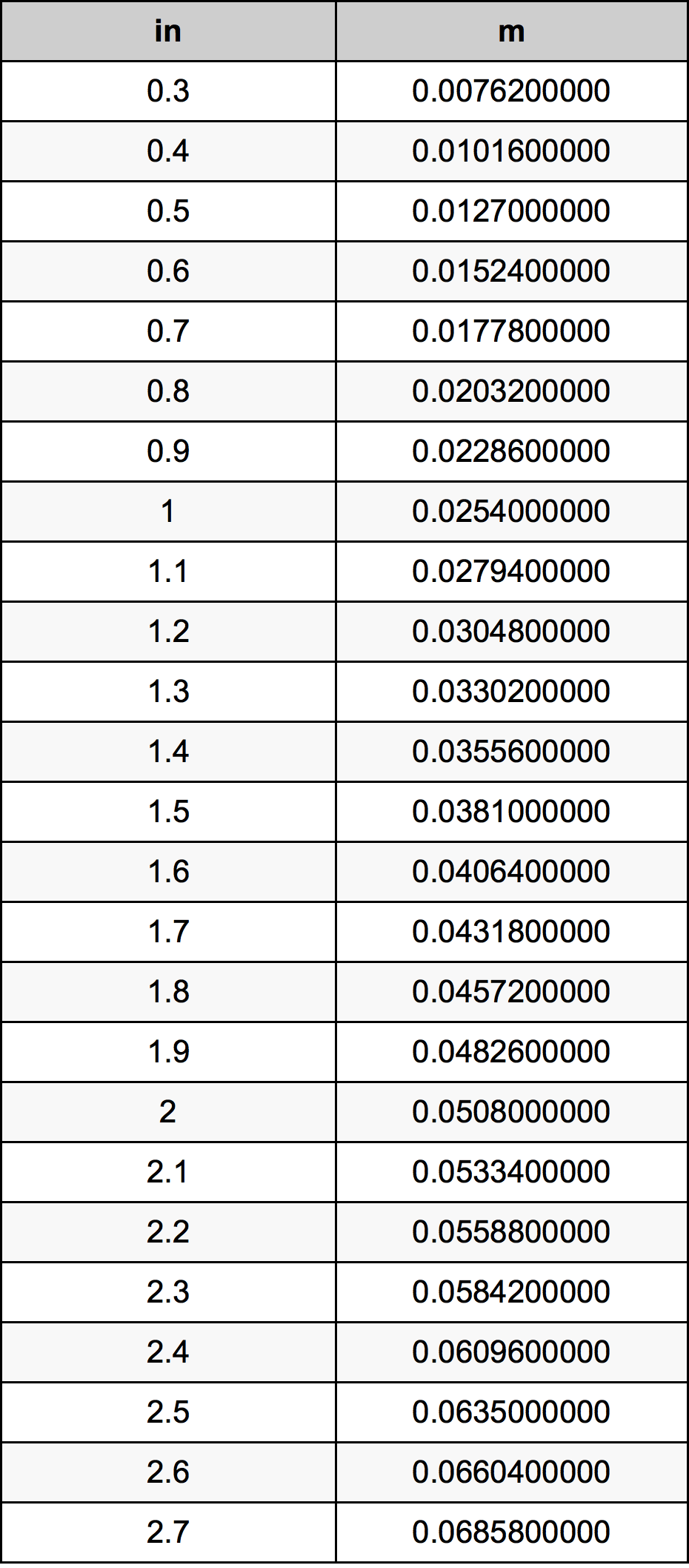Inches To Meters

# 1.5 in to m1.5 Inches to Meters

in
=
m

## How to convert 1.5 inches to meters?

 1.5 in * 0.0254 m = 0.0381 m 1 in
A common question is How many inch in 1.5 meter? And the answer is 59.0551181102 in in 1.5 m. Likewise the question how many meter in 1.5 inch has the answer of 0.0381 m in 1.5 in.

## How much are 1.5 inches in meters?

1.5 inches equal 0.0381 meters (1.5in = 0.0381m). Converting 1.5 in to m is easy. Simply use our calculator above, or apply the formula to change the length 1.5 in to m.

## Convert 1.5 in to common lengths

UnitUnit of length
Nanometer38100000.0 nm
Micrometer38100.0 µm
Millimeter38.1 mm
Centimeter3.81 cm
Inch1.5 in
Foot0.125 ft
Yard0.0416666667 yd
Meter0.0381 m
Kilometer3.81e-05 km
Mile2.36742e-05 mi
Nautical mile2.05724e-05 nmi

## What is 1.5 inches in m?

To convert 1.5 in to m multiply the length in inches by 0.0254. The 1.5 in in m formula is [m] = 1.5 * 0.0254. Thus, for 1.5 inches in meter we get 0.0381 m.

## 1.5 Inch Conversion Table## Alternative spelling

1.5 in to Meter, 1.5 in in Meter, 1.5 Inch to Meter, 1.5 Inch in Meter, 1.5 in to m, 1.5 in in m, 1.5 Inch to m, 1.5 Inch in m, 1.5 Inches to Meter, 1.5 Inches in Meter, 1.5 Inches to Meters, 1.5 Inches in Meters, 1.5 Inch to Meters, 1.5 Inch in Meters